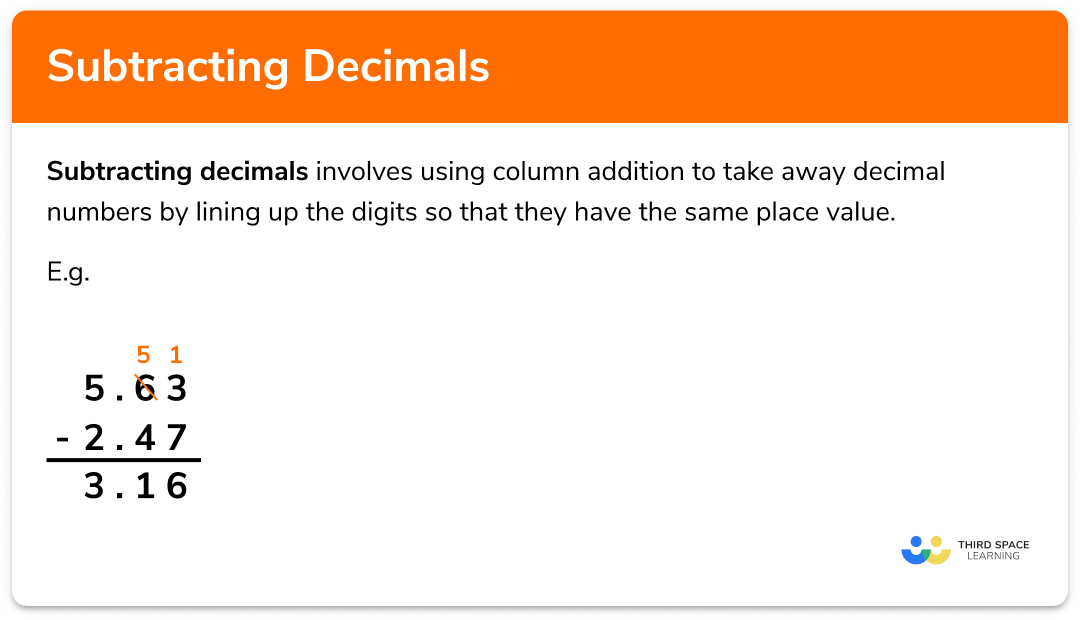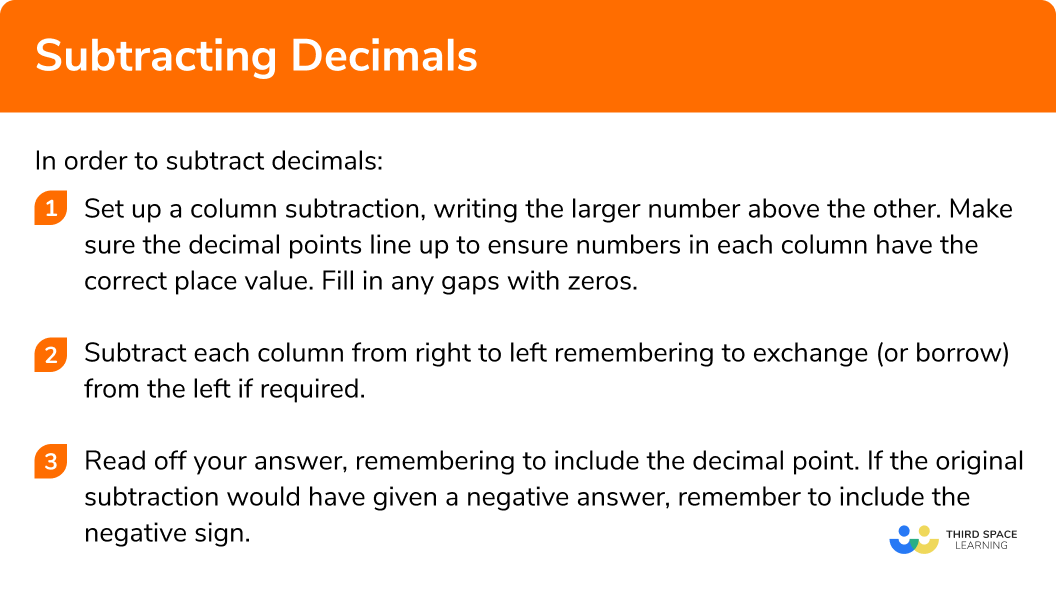# Subtracting Decimals

Here we will learn about subtracting decimals including how to solve problems that involve the subtraction of decimal numbers.

There are also subtracting decimals worksheets with word problems and problem solving questions based on Edexcel, AQA and OCR exam questions, along with further guidance on where to go next if you’re still stuck.

## What is subtracting decimals?

Subtracting decimals involves using column addition to subtract decimal numbers by lining up the digits so that they have the same place value.

The ones, decimal points, tenths, hundredths etc. should be lined up. This is particularly important to do if the values we are adding have been given to a different number of decimal places.

This column method of subtraction only works if the number we are subtracting is smaller than the one we are subtracting it from. If we know our answer will be negative, we must switch the numbers around, find the positive difference and then make our answer negative.

### What is subtracting decimals?## How to subtract decimals

In order to subtract decimals:

1. Set up a column subtraction, writing the larger number above the other. Make sure the decimal points line up to ensure numbers in each column have the correct place value. Fill in any gaps with zeros.
2. Subtract each column from right to left remembering to exchange (or borrow) from the left if required.
3. Read off your answer, remembering to include the decimal point. If the original subtraction would have given a negative answer, remember to include the negative sign.

### How to subtract decimals### Related lessons on decimals

Subtracting decimals is part of our series of lessons to support revision on decimals. You may find it helpful to start with the main decimals lesson for a summary of what to expect, or use the step by step guides below for further detail on individual topics. Other lessons in this series include:

## Subtracting decimals examples

### Example 1: Subtracting numbers with one decimal place

Work out 7.32.8.

1. Set up a column subtraction, writing the larger number above the other. Make sure the decimal points line up to ensure numbers in each column have the correct place value. Fill in any gaps with zeros.

2Subtract each column from right to left remembering to exchange (or borrow) from the left if required.

3Read off your answer, remembering to include the decimal point. If the original subtraction would have given a negative answer, remember to include the negative sign.

### Example 2: subtracting numbers with two decimal places

Work out 12.437.81.

### Example 3: subtracting numbers with different amounts of decimals places

Work out 9.13.276.

### Example 4: subtracting larger decimal numbers from smaller decimal numbers

Work out 4.367.21.

This subtraction will give a negative answer. We will need to swap the order to make the column subtraction method work. So we will work out 7.214.36, and make our answer negative later.

We swapped the order of the numbers in our subtraction so we need to make our answer negative.

### Example 5: subtracting more than one decimal number from another

Find the change from £20 if items costing £3.47 and £11.79 are purchased.

We could do two separate subtractions or add the two items to be subtracted and subtract the total from £20.

Here we will add the two items and then set up one subtraction.

### Common misconceptions

• Not lining up the decimal point

A common error when subtracting decimals occurs when the decimal points are not lined up but instead the last digits are lined up.

E.g.

• Not swapping the order when taking a larger number from a smaller number

It is important to remember that the column subtraction method does not give the correct answer if it is used with a smaller number above a larger number. If it is clear that the answer will be negative, we must remember to swap the order of the numbers for the subtraction and then make the answer negative.

### Practice subtracting decimals questions

1. Work out 12.5  −  7.8

4.75.320.35.7You must remember to line up the decimal points carefully and exchange (or borrow) from the left if required.2. Work out 56.29  −  2.47

 3.15931.5953.8254.22You must remember to line up the decimal points carefully and exchange (or borrow) from the left if required.3. Work out 23.4  −  16.573

16.3397.1736.8277.827You must remember to line up the decimal points carefully, filling in any gaps with zeros. Exchange (or borrow) from the left if required.4. Work out 3.51  −  8.29

4.78-5.325.32-4.78You must remember to swap the order of the numbers to perform column subtraction, then put a negative sign on the answer.5. Work out 8.5  −  1.4  −  5.9

41.22.82Either add 1.4 and 5.9 together first and then subtract from 8.5 , or subtract 1.4 from 8.5 and then 5.9 from the answer.

6. Work out 11.2  −  3.6  −  2.01

9.615.56.135.59Either add 3.6 and 2.01 together first and then subtract from 11.2 , or subtract 3.6 from 11.2 and then 2.01 from the answer.

### Subtracting decimals GCSE questions

1.

a) Work out 12.79  −  5.82

b) Work out 44.6  –  23.39  –  8.42

(3 marks)

a)

(1)

b)

21.21 or 36.18 or 31.81 seen

(1)

(1)

2. Complete the following calculations:

a)b)(5 marks)

a)

9 in the top row.

(1)

3 in the second row.(1)

b)

9 in first box in top row.

(1)

4 in second box in top row.

(1)

8 in second row.(1)

3. Mark went to a clothes shop and bought 2 t-shirts costing £8.99 each, a hat costing £6.50 and a pair of shoes costing £22.90.

He paid for the items with a £50 note.

(3 marks)

£8.99 + £8.99 + £6.50 + £22.90

(1)

£50  –  £47.38.

(1)

(1)

## Learning checklist

You have now learned how to:

• Understand and use place value for decimals, measures and integers of any size
• Use the four operations, including formal written methods, applied to integers, decimals, proper and improper fractions, and mixed numbers, all both positive and negative

## Still stuck?

Prepare your KS4 students for maths GCSEs success with Third Space Learning. Weekly online one to one GCSE maths revision lessons delivered by expert maths tutors.

Find out more about our GCSE maths tuition programme.